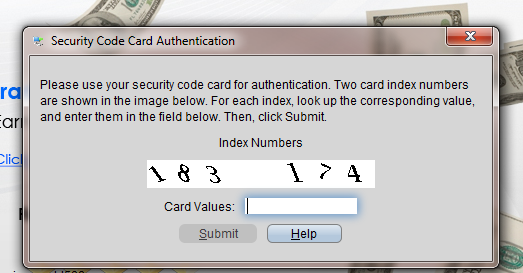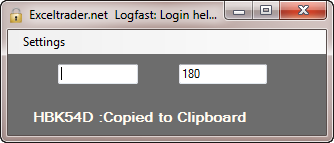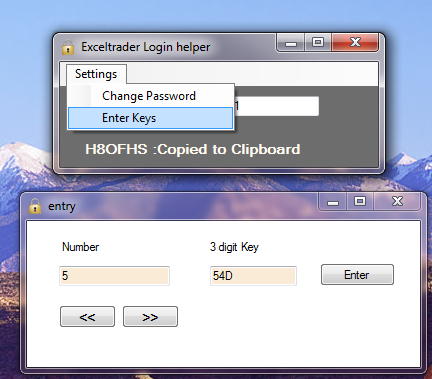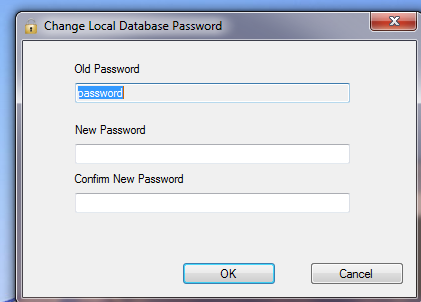## A small program to assist IB login for users with Security cards.

IB now provides account holders with a plastic security card that has 224 numbers (1-224)  listed next to each number’s corresponding three digit unique key. To login, the user must look up two numbers provided by IB at login and then find and type in the corresponding two keys. Many find the process cumbersome and opt out of being required to use the security device. Opting out requires the account holder to sign forms giving up certain rights and is not a great choice considering that IB for some reason limits password maximum length to 8 characters.LogFast_et is a small program to assist the cumbersome process of logging in. Once the program is set up, The user opens the small program, enters the 2 numbers displayed by IB and the resulting code is copied automatically to the clipboard so that it can be pasted in  IB’s login area to complete the login.

Initial Setup: Download and install the program. To setup the program, there is a form in place available from the settings menu to enter the values from the security card. The setup should take most people around 20 minutes to enter all 224 entries. Fortunately you’ll only have to do it once.Below is a short video showing the program in action.  Please use the comment form below for any questions, comments, feature requests.

## Stochastics Indicator – Excel VBA and Formula versions

This post includes  two example excel files that show both VBA and Formula based calculations for the indicator "Stochastics".

Both files contain the exact same set of Open High Low Close data. The formula based version should be easier to understand and serves as a way to verify that the VBA code that I wrote is correct. Both methods give the same result for both %k and %D.  The major benefit of using VBA is that the parameters for Stochastics can be easily changed from the input boxes. In addition the VBA method shows only the final result rather than take up five columns.

The formula version can be downloaded here. Below is an image of the formulas used in the formula based version.Below is the code that I wrote for the VBA based version.

Dim StochSetting As Integer, Ksetting As Integer, Dsetting As Integer
Dim A() As Double, B() As Double, C() As Double, D() As Double, E() As Double
Dim Count As Long
Dim Xcounter As Integer, Xavg As Double
Dim Zcounter As Integer, Zavg As Double
Dim x As Integer, y As Integer, z As Integer
'''''''''''''''''''''''''''''''''''''''''''''''''''''''''''''''''''''''''''''''''''''''''''
''  The below three lines are the Stochastic settings.
''  The Values can either be changed here or uncomment the inputbox lines to be prompted.
'''''''''''''''''''''''''''''''''''''''''''''''''''''''''''''''''''''''''''''''''''''''''''
StochSetting = 14 'InputBox(Prompt:="Enter Stoch settings Number of Periods.", Title:="Stochastic Period", Default:="13")
Ksetting = 2 'InputBox(Prompt:="Enter Moving Average For %K", Title:="%K Setting", Default:="5")
Dsetting = 3 'InputBox(Prompt:="Enter Moving Average For %D", Title:="%D Setting", Default:="6")
Set ws = ThisWorkbook.Worksheets("Svba")
With ws
LR = .Cells(Rows.Count, "A").End(xlUp).Row
'does the same as formula =E15-(MIN(D1:D15))
For Each DataRange In ws.Range(.Cells(2, "A"), .Cells(LR, "A"))
Count = DataRange.Row
ReDim Preserve A(1 To Count)
If Count >= StochSetting + 1 Then
A(Count) = .Cells(Count, "E") - Application.Min(ws.Range(.Cells(Count - StochSetting, "D") _
, .Cells(Count, "D")))
End If
Next DataRange
'does the same as formula   =MAX(C36:C50)-MIN(D36:D50)
For Each DataRange In ws.Range(.Cells(2, "A"), .Cells(LR, "A"))
Count = DataRange.Row
ReDim Preserve B(1 To Count)
If Count >= StochSetting + 1 Then
B(Count) = Application.Max(ws.Range(.Cells(Count - StochSetting, "C"), .Cells(Count, "C"))) _
- Application.Min(ws.Range(.Cells(Count - StochSetting, "D"), .Cells(Count, "D")))
End If
Next DataRange
'=100*(I50/J50)
ReDim Preserve C(StochSetting + 1 To UBound(B))
For Count = StochSetting + 1 To UBound(B)
C(Count) = (A(Count) / B(Count)) * 100
Next Count
'=AVERAGE(K49:K50)
ReDim Preserve D(StochSetting + Ksetting To UBound(B))
For Count = StochSetting + Ksetting To UBound(B)
For Xcounter = Count - Ksetting + 1 To Count 'just go back and get the first C and go forward to current
Xavg = C(Xcounter) + Xavg
Next Xcounter
D(Count) = Xavg / Ksetting
Xavg = Empty
Next Count
ReDim Preserve E(StochSetting + Ksetting + Dsetting - 1 To UBound(B))
For Count = StochSetting + Ksetting + Dsetting - 1 To UBound(B)
For Zcounter = Count - Dsetting + 1 To Count 'just go back and get the first C and go forward to current
Zavg = D(Zcounter) + Zavg
Next Zcounter
E(Count) = Zavg / Dsetting
Zavg = Empty
Next Count
x = Empty: y = Empty: z = Empty
x = LBound(E)
y = UBound(E)
'put the stochastics on the workbook. Change column Letter as needed
.Cells(x - 1, "J") = "%D"
.Cells(x - 1, "I") = "%K"
For z = x To y
.Cells(z, "J") = E(z)
.Cells(z, "I") = D(z)
Next z
End With
End Sub

## MACD VBA

This post includes links to two example xls files that show both VBA and Formula based calculations for the indicator MACD or Moving Average Convergence Divergence.

Both files contain the exact same set of Open High Low Close data. The formula based version is easier to understand and serves as a way to verify that the VBA code that I wrote is correct. Both methods give the same result.  The major benefit of using VBA is that the parameters for MACD can be easy changed from the input boxes. In addition the VBA method shows only the final result rather than take up five columns.

The formula version can be downloaded here. Below is the code from the VBA based version.

Dim EMAslow As Double, EMAfAst As Double, ws As Worksheet, LR As Integer
Dim eMaF() As Double, eMaS() As Double, EMAdif(), emaPer() As Double, MacDper As Double, coUnt As Integer
Dim DataRange As Range
Dim ExPSlowWeight As Double
Dim ExPFastWeight As Double
Dim PerWeight As Double
'''''''''''''''''''''''''''''''''''''''''''''''''''''''''''''''''''''''''''''''''''''''''''
''  The below three lines are the MACD settings.                                         ''
''  The Values can either be changed here or uncomment the inputbox lines to be prompted.''
'''''''''''''''''''''''''''''''''''''''''''''''''''''''''''''''''''''''''''''''''''''''''''
EMAslow = 13 'InputBox(Prompt:="Enter Macd Slow settings.", Title:="MACD SLOW", Default:="13")
EMAfAst = 5 'InputBox(Prompt:="Enter Macd Fast settings.", Title:="MACD Fast", Default:="5")
MacDper = 6 'InputBox(Prompt:="Enter Macd Period settings.", Title:="MACD Period", Default:="6")
ExPSlowWeight = 2 / (EMAslow + 1)
PerWeight = 2 / (MacDper + 1)
ExPFastWeight = 2 / (EMAfAst + 1)
Set ws = ThisWorkbook.Worksheets("VBA") 'or use exact sheet name for example ThisWorkbook.worksheet("Sheet1")
'slow
With ws
LR = .Cells(Rows.coUnt, "A").End(xlUp).Row
For Each DataRange In ws.Range(.Cells(2, "A"), .Cells(LR, "A"))
coUnt = DataRange.Row + 1
'fill the eMA slow Array
ReDim Preserve eMaS(1 To coUnt)
If coUnt = EMAslow + 1 Then
'get the first value which is the Simple Moving average
eMaS(coUnt) = Application.Average(ws.Range(.Cells(2, "E"), .Cells(coUnt, "E")))
ElseIf coUnt > EMAslow Then
eMaS(coUnt) = (.Cells(coUnt, "E") * ExPSlowWeight) + (eMaS(coUnt - 1) * (1 - ExPSlowWeight))
End If
Next DataRange
'fast
For Each DataRange In ws.Range(.Cells(2, "A"), .Cells(LR, "A"))
coUnt = DataRange.Row + 1
'fill the eMA slow Array
ReDim Preserve eMaF(1 To coUnt)
If coUnt = EMAfAst + 1 Then
'get the first value which is the Simple Moving average
eMaF(coUnt) = Application.Average(ws.Range(.Cells(2, "E"), .Cells(coUnt, "E")))
ElseIf coUnt > EMAfAst Then
eMaF(coUnt) = (.Cells(coUnt, "E") * ExPFastWeight) + (eMaF(coUnt - 1) * (1 - ExPFastWeight))
End If
Next DataRange
For coUnt = EMAslow + 1 To UBound(eMaF)
Next coUnt
'MacD Period
Dim x As Integer, y As Integer, z As Integer, Avee As Double
y = EMAslow + MacDper - 1
For x = y To UBound(EMAdif) - 2
'get the SMA for first value
If x = y Then
For z = EMAslow + 1 To EMAslow + MacDper  '(EMAslow + MacDper - 1)
Next z
ReDim emaPer(x To LR)
emaPer(x) = Ave / MacDper
ElseIf x > y Then
emaPer(x) = (EMAdif(x + 1) * PerWeight) + (emaPer(x - 1) * (1 - PerWeight))
End If
Next x
x = Empty: y = Empty: z = Empty
x = LBound(emaPer)
y = UBound(emaPer)
For z = x To y - 1
.Cells(z + 1, "J") = EMAdif(z + 1) - emaPer(z)
Next z
End With

## Data Converter to Convert OHLC Data to a larger timeframe.

If you have ever wanted to take your existing Open High Low Close historical trading data and convert it into larger time frame, the following Excel VBA based data converter I wrote may do the trick.DataConverter.xls allows you to configure the Number of OHLC bars that should be condensed down into a single bar. You also can list the file name, sheet, and columns where your data is located.  The data will almost instantly be transformed into the higher time frame you have requested.

Instructions: